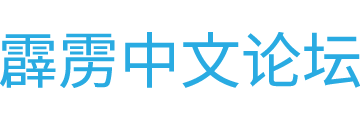# 【韩语教室】关于韩国字母

1446年，韩国出现了最早的表音文字－训民正音。其含义正如它的名字所示，是“纠正国民的发音”之意。韩字的创始人世宗大王是韩国历史上非常杰出的一位君王，他心胸宽广，执政勤勉， 为世人所尊崇。他不仅是一位成功的政治家，更还是一位极富激情的学者，他的才能和学识令很多学者惊叹不已。

ㄱ(g,k)
ㄴ(n)
ㄷ(d,t)
ㄹ(r or l)
ㅁ(m)
ㅂ(b,p)
ㅅ(s)
ㅇ(ng)
ㅈ(j)
ㅊ(ch)
ㅋ(k)
ㅌ(t)
ㅐ §
ㅎ(h)

ㅏ(a)
ㅑ (ya)
ㅓ (eo)
ㅕ(yeo)
ㅗ(o)
ㅛ(yo)
ㅜ(u)
ㅠ(yu)
ㅡ(eu)
ㅣ(i)

[b]ㄱ+ㅏ=가(ga)
ㄱ+ㅑ=갸(gya)
ㄱ+ㅓ=거(geo)
ㄱ+ㅕ=겨(gyeo)
ㄱ+ㅗ=고(go)
ㄱ+ㅛ=교(gyo)
ㄱ+ㅜ=구(gu)
ㄱ+ㅠ=규(gyu)
ㄱ+ㅡ=그(geu)
ㄱ+ㅣ=기(gi)

ㄴ+ㅏ=나(na)
ㄴ+ㅑ=냐(nya)
ㄴ+ㅓ=너(neo)
ㄴ+ㅕ=녀(nyeo)
ㄴ+ㅗ=노(no)
ㄴ+ㅛ=뇨(nyo)
ㄴ+ㅜ=누(nu)
ㄴ+ㅠ=뉴(nyu)
ㄴ+ㅡ=느(neu)
ㄴ+ㅣ=니(ni)[/b]

＠＠韩文我不是很喜欢。。

doitashimashte`

huh??? @_@??

pening。。。。。。pening

=)